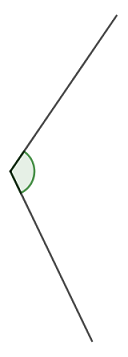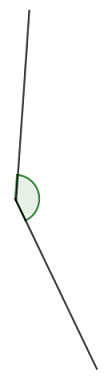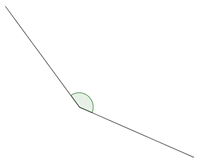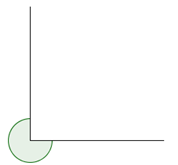# Shapes and Angles

## Objective

Use iteration with one physical unit to measure benchmark angles.

## Common Core Standards

### Core Standards

?

• 4.MD.C.5 — Recognize angles as geometric shapes that are formed wherever two rays share a common endpoint, and understand concepts of angle measurement:

• 4.MD.C.6 — Measure angles in whole-number degrees using a protractor. Sketch angles of specified measure.

## Criteria for Success

?

1. Directly compare the size of two angles.
2. Understand angle measure as the spread of an angle’s rays.
3. Use the understanding that the size of an angle is determined by how far apart its rays are spread to create a nonstandard unit, a wedge, to measure them (MP.7).
4. Measure angles with a nonstandard unit (a “wedge”) by iterating a single unit with no gaps or overlaps.

## Tips for Teachers

?

• For the paper circles, you can use parchment paper baking circles. They are easy to find online or at restaurant supply stores in large quantities.
• Students will create a wedge template using a paper circle in this lesson. Have students save this template for future lessons.
• Make sure students hold on to Problem Sets for the next day, since they will come back to them in Lesson 6.
• Students will need their wedges to measure angles on the Homework. If you do not want students to bring them home, the Homework can be cut.
• If at any point in the lesson students suggest unfolding their "wedges" to measure the angles throughout the lesson, allow those students to do so. Otherwise, wait until Lesson 6 to unfold the wedges, when they will create their own makeshift protractors that measure in degrees.
• John A. Van de Walle notes, "angle measurement can be a challenge for two reasons: the attribute of angle size is often misunderstood, and protractors are commonly introduced and used without understanding how they work" (Teaching Student-Centered Mathematics: Developmentally Appropriate Instruction for Grades 3-5 (Volume II). Pearson, 2nd edition, 2013. Page 335). Thus, this lesson serves to help students understand angle measure as the spread of an angle’s rays, which can be measured with smaller angles.

### Lesson Materials

• Paper circle (1 per student)

#### Fishtank Plus

• Problem Set
• Student Handout Editor
• Vocabulary Package

?

### Problem 1

Which angle is larger? How do you know?

 a.b.### Problem 2

Fold a circular piece of paper in half, then in half again. How big is the angle that you created? How could you create a smaller angle to use as a unit to measure angle size?

### Problem 3

Measure the angles in Anchor Task #1 in wedges.

## Problem Set & Homework

### Discussion of Problem Set

• Make sure students hold on to Problem Sets for the next day, since they will come back to them in Lesson 6.
• If someone asked you to define what an angle is, what would you say? If someone asked you how they could measure one, what would you say?
• Look at #2(e). What made this difficult to measure? What did you get?
• Look at #2(f). What made this difficult to measure? What did you get?
• Look at #4. If you used an even smaller wedge, would it give you a more or less accurate way to measure the size of an angle?

?

Use your wedge to measure the angles below.

a.b.#### Mastery Response